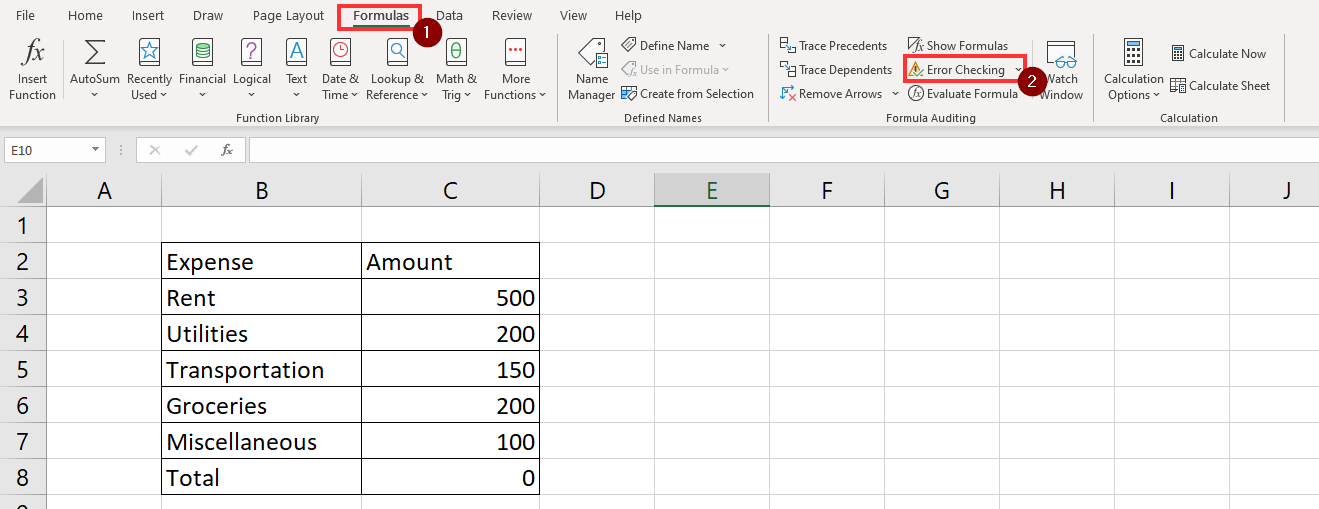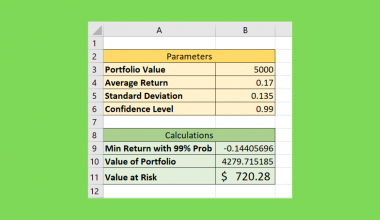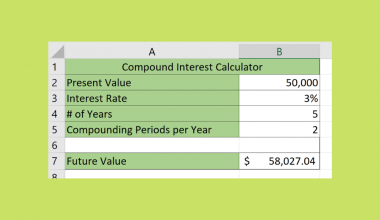# Fixing Circular References that Cannot Be Listed in Excel

This guide will explain how to fix circular references that are not listed in Excel.

We’ll list down a few methods you can use to find circular references in your spreadsheet. For circular references that are not listed, we can also edit the Excel calculation settings to settle the values.

Let’s first understand why circular references happen in your spreadsheet.

Suppose you have to find the sum of a range of values. We can use the SUM function to calculate the total in a range.

What happens if the cell containing the SUM function is included in the given range? If the formula is set up this way, it will lead to an endless loop of calculations. Because it is impossible to evaluate an endless loop, Excel flags these formulas as a circular reference.

Most circular references are caught by Excel’s Error Checking feature. Later in the article, we’ll show you how to find circular references using this feature.

Excel returns a value of 0 by default. However, you can choose to set a certain number of iterations to allow before Excel evaluates the function.

Changing the number of allowable iterations is the best way to fix circular references in Excel.

Now that we know when to fix circular references, let’s dive into how to use it and work on an actual sample spreadsheet.

## A Real Example of a Circular Reference in Excel

In the following section, we will provide a few examples of circular references in Excel. We’ll also go in-depth on the formulas and tools used in these examples.

First, let’s take a look at a real example of a circular reference in an Excel spreadsheet.

The table below contains data on various expenses and their corresponding amount. Cell C8 tries to provide the total amounts of each expense category. The cell returns a 0 rather than the actual total.Notice how the SUM function uses the range C3:C8 accidentally rather than C3:C7. The accidental input leads to a circular reference.

We can use the Error Checking tool to list circular references in our spreadsheet.However, it is possible for some circular references to not appear in this drop-down menu.

To fix these issues, we suggest editing the calculation settings of your Excel program. In the next section, we’ll show an example of how to do this yourself.

Do you want to take a closer look at our examples? You can make your own copy of the spreadsheet above using the link attached below.

If you’re ready to fix circular references that cannot be listed in Excel, head over to the next section to read our step-by-step breakdown on how to do it!

## How to Fix Circular Reference that Cannot Be Listed in Excel

This section will guide you through each step needed to fix circular references in Excel. You’ll learn how we can allow iterative calculations to let circular references evaluate after a certain number of steps.

Follow these steps to start fixing unlisted circular references:

1. We can use the status bar to check at a glance if there are circular references in the current spreadsheet. In the example below, the status bar indicates that cell C8 has a circular reference. A downside to this method is that it only detects the most recent circular reference.2. We can also use the Error Checking feature to look for circular references. In the Formulas tab, click on the Error Checking option.3. In the dropdown menu, hover over the Circular References option to see a list of found circular references.4. Clicking on any of these cell references will take your cursor to the referenced cell.5. We can also handle all circular references by changing your Excel program’s calculation options. First, click on the File tab on the top left corner of your screen.6. Click on the Options button on the page’s lower-left side.7. In the Excel Options dialog menu, navigate to the Formulas tab and look for the section labeled ‘Calculation options’. Check the checkbox for the option labeled ‘Enable iterative calculation’. For the ‘Maximum iterations’ input, you may place your desired number of iterations to run before Excel returns the output.These are all the steps you need to fix all circular references in your Excel spreadsheet.

This step-by-step guide should be all you need to fix unlisted circular references in Excel yourself. Our guide shows how easy it is to change Excel calculation options to allow iterative calculations.

Iterative calculations are one way to modify your sheet to work with your dataset. Our website covers hundreds of other Excel methods and functions that you can apply to your own spreadsheets.

We have dozens of different guides you can use to fix problems you encounter in your spreadsheet. For example, you can check out this guide if your formulas are not updating properly.

With so many other Excel functions available, you can surely find one that suits your use case.

Want to learn more about what Excel can do? Subscribe to our newsletter to look into the latest Excel guides and tutorials from us.### Get emails from us about Excel.

Our goal this year is to create lots of rich, bite-sized tutorials for Excel users like you. If you liked this one, you'd love what we are working on! Readers receive ✨ early access ✨ to new content.

##### You May Also Like## How to Perform a VLOOKUP with Time Range in Excel

This guide will explain how you can use VLOOKUP with a given time range in Excel. We can…## How to Create Value at Risk Template in Excel

A Value at Risk template in Excel is useful when you need to find out the potential loss…## How to Find Circular References in Excel

This guide will explain how you can use Excel’s Error Checking feature to find circular reference errors in…## How to Use Compound Interest Formula in Excel

ForssThe FV function in Excel is useful when you need to use the compound interest formula to determine…